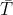×
Get Full Access to Statistics For Engineers And Scientists - 4 Edition - Chapter 7.2 - Problem 16e
Get Full Access to Statistics For Engineers And Scientists - 4 Edition - Chapter 7.2 - Problem 16e

×

# A mixture of sucrose and water was heated on a hot plate,ISBN: 9780073401331 38

## Solution for problem 16E Chapter 7.2

Statistics for Engineers and Scientists | 4th Edition

• Textbook Solutions
• 2901 Step-by-step solutions solved by professors and subject experts
• Get 24/7 help from StudySoup virtual teaching assistantsStatistics for Engineers and Scientists | 4th Edition

4 5 1 332 Reviews
23
1
Problem 16E

A mixture of sucrose and water was heated on a hot plate, and the temperature (in °C) was recorded each minute for 20 minutes by the three thermocouples.a. Compute the least-squares line for estimating the temperature as a function of time, using T1 as the value for temperature.

b. Compute the least-squares line for estimating the temperature as a function of time, using T2 as the value for temperature.

c. Compute the least-squares line for estimating the temperature as a function of time, using T3 as the value for temperature.

d. It is desired to compute a single line to estimate temperature as a function of time. One person suggests averaging the three slope estimates to obtain a single slope estimate and averaging the three intercept estimates to obtain a single intercept estimate. Find the equation of the line that results from this method.

e. Someone else suggests averaging the three temperature measurements at each time to obtain= (T1 + T2 + T3)/3. Compute the least-squares line usingas the value for temperature.

f. Are the results of parts (d) and (e) different?

Step-by-Step Solution:
Step 1 of 3

GEOL 207 Environmental Geology More Atmosphere Stuff!! - Albedo  reflection of light on earth. o Clouds  - Air and atmosphere in a system o Circular system (atmosphere)  Much faster than other circular systems such as plate tectonics. - CIRCULAR SYSTEMS (air) o Energy comes from the sun o Buoyancy  The ability or tendency to float in water or air or some other fluid.  Factors  Pressure  Volume  Temperature  If factor (variable) is constant, you can omit it from the equation - Lateral Movement o Sideways movement

Step 2 of 3

Step 3 of 3

##### ISBN: 9780073401331

The answer to “?A mixture of sucrose and water was heated on a hot plate, and the temperature (in °C) was recorded each minute for 20 minutes by the three thermocouples. a. Compute the least-squares line for estimating the temperature as a function of time, using T1 as the value for temperature.b. Compute the least-squares line for estimating the temperature as a function of time, using T2 as the value for temperature.c. Compute the least-squares line for estimating the temperature as a function of time, using T3 as the value for temperature.d. It is desired to compute a single line to estimate temperature as a function of time. One person suggests averaging the three slope estimates to obtain a single slope estimate and averaging the three intercept estimates to obtain a single intercept estimate. Find the equation of the line that results from this method.e. Someone else suggests averaging the three temperature measurements at each time to obtain = (T1 + T2 + T3)/3. Compute the least-squares line using as the value for temperature.f. Are the results of parts (d) and (e) different?” is broken down into a number of easy to follow steps, and 180 words. This textbook survival guide was created for the textbook: Statistics for Engineers and Scientists , edition: 4. The full step-by-step solution to problem: 16E from chapter: 7.2 was answered by , our top Statistics solution expert on 06/28/17, 11:15AM. This full solution covers the following key subjects: temperature, line, compute, squares, least. This expansive textbook survival guide covers 153 chapters, and 2440 solutions. Statistics for Engineers and Scientists was written by and is associated to the ISBN: 9780073401331. Since the solution to 16E from 7.2 chapter was answered, more than 381 students have viewed the full step-by-step answer.

Unlock Textbook Solution